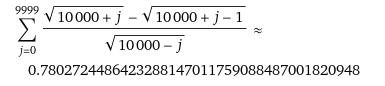# Proving this equation -- Limit of a sum of inverse square root terms

alsdt
Homework Statement:
calculate the limit of a phrase
Relevant Equations:
limit
Hi
I was working on a physics problem and it was almost solved.
Only the part that is mostly mathematical remains, and no matter how hard I tried, I could not solve it.
I hope you can help me.
This is the equation I came up with and I wanted to prove it: $$\lim_{n \rightarrow+ \infty} { \sum_{i=1}^n \frac 1 {\sqrt{i(2n-i-1)} +\sqrt{i(2n-i)}}}=\frac \pi 4$$

•Delta2

Homework Helper
Gold Member
What have you tried?
Can you show some work?
What is the context? It might provide some insight.

Staff Emeritus
Gold Member
I don't have a proof, but typically you have to turn these types of things into a Riemann sum. I think you can probably argue the -1 in the one term is irrelevant, at which point

https://www.wolframalpha.com/input/?i=int_0^1+1/sqrt(x(1-x))+dx

Is pretty suggestive

•PeroK and mfb
Gold Member
Wolfram showsSo you seem to be on right track.

•PeroK and robphy
Gold Member
As shown in post #4 you may transform the sum as
$$\sum_x \frac{\sqrt{1+x}-\sqrt{1+y}}{\sqrt{1-x}}$$
where x = ##{0,\ 1/n,\ 2/n,\ ...\ , (n-1)\ /n}## and y= x - 1/n
, which is convenient for transformation to integral.

Last edited:
Homework Helper
Gold Member
Wolfram shows
View attachment 291038

So you seem to be on right track.

As shown in post #4 you may transform the sum as
$$\sum_x \frac{\sqrt{1+x}-\sqrt{1+y}}{\sqrt{1-x}}$$
where x = ##{0,\ 1/n,\ 2/n,\ ...\ , (n-1)\ /n}## and y= x - 1/n
, which is convenient for transformation to integral.
Thanks for editing and adding details (like what y is).
On its own, Post #4 says that Wolfram shows [something], but it wasn't clear how or what is being shown.

Are you saying that Wolfram transformed the original series into what is shown in Post #4?

Gold Member
Are you saying that Wolfram transformed the original series into what is shown in Post #4?
No, I did it by myself in a usual way.

Last edited: